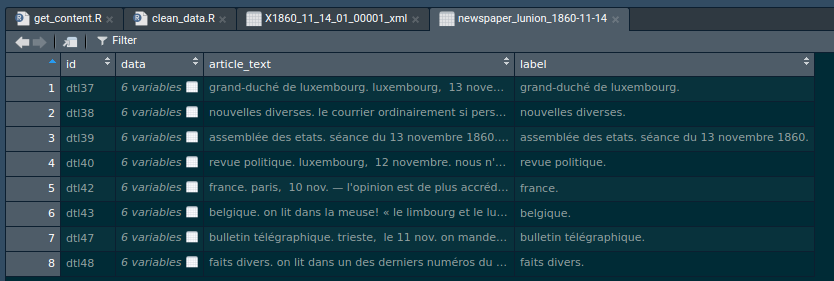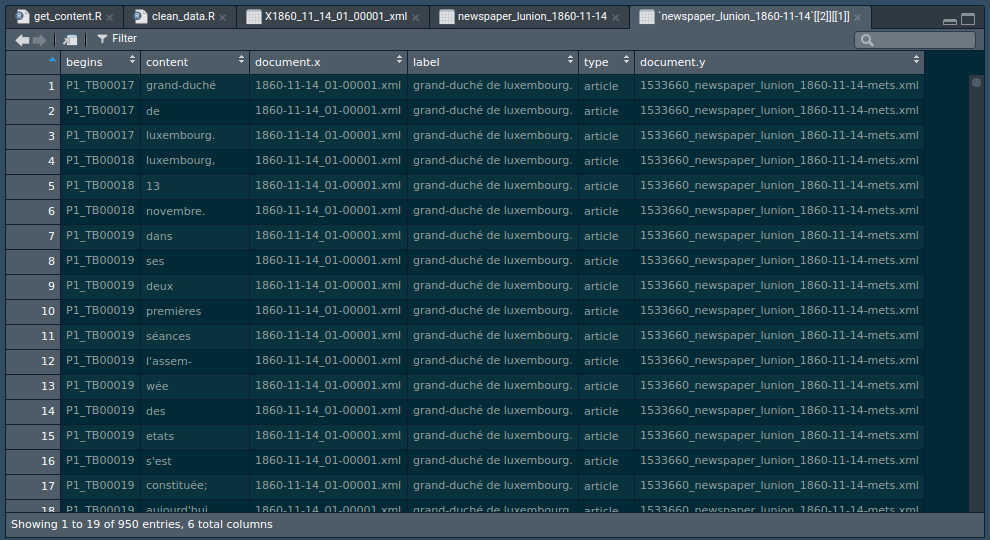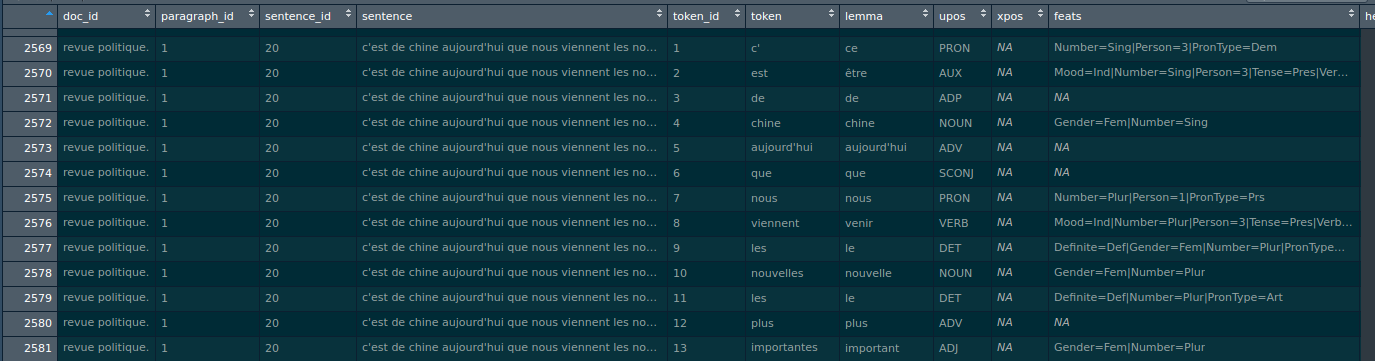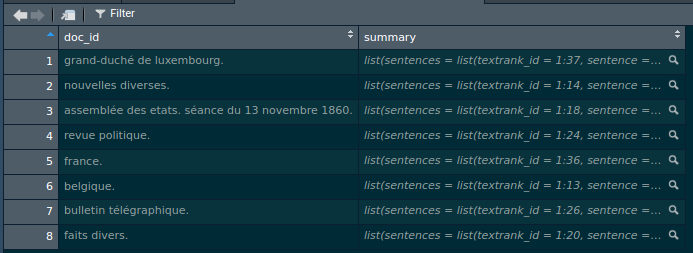Have a question about one of the blog posts? Chat here with me.

# Building a shiny app to explore historical newspapers: a step-by-step guide

R

## Introduction

I started off this year by exploring a world that was unknown to me, the world of historical newspapers. I did not know that historical newspapers data was a thing, and have been thoroughly enjoying myself exploring the different datasets published by the National Library of Luxembourg. You can find the data here.

In my first blog post, I analyzed data from L’indépendence Luxembourgeoise. I focused on the ads, which were for the most part in the 4th and last page of the newspaper. I did so by extracting the data from the ALTO files. ALTO files contain the content of the newspapers, (basically, the words that make up the article). For this first exercise, I disregarded the METS files, for two reasons. First, I simply wanted to have something quick, and get used to the data. And second, I did not know about ALTO and METS files enough to truly make something out of them. The problem of disregarding the METS file is that I only had a big dump of words, and did not know which words came from which article, or ad in this case.

In the second blog post), I extracted data from the L’Union newspaper, this time by using the metadata from the METS files too. By combining the data from the ALTO files with the metadata from the METS files, I know which words came from which article, which would make further analysis much more interesting.

In the third blog post of this series, I built a Shiny app which makes it easy to explore the 10 years of publications of L’Union. In this blog post, I will explain in great detail how I created this app.

## Part 1: Getting the data ready for the Shiny app

### Step 1: Extracting the needed data

If you want to follow along with a dataset from a single publication, you can download the following archive on dropbox. Extract this archive, and you will find the data exactly as you would get it from the the big archive you can download from the website of the National Library of Luxembourg. However, to keep the size of the archive small, I removed the .pdf and .jpeg scans.

In the second blog post) I wrote some functions that made extracting the needed data from the files easy. However, after I wrote the article, I noticed that in some cases these functions were not working exactly as intended. I rewrote them a little bit to overcome these issues. You can find the code I used right below. I won’t explain it too much, because you can read the details in the previous blog post. However, should something be unclear, just drop me an email or a tweet!

Click if you want to see the code

``````# This functions will be used within the next functions to extract the relevant pieces

extractor <- function(string, regex, all = FALSE){
if(all) {
string %>%
str_extract_all(regex) %>%
flatten_chr() %>%
str_remove_all("=|\\\"") %>%
#str_extract_all("[:alnum:]+|.|,|\\?|!", simplify = FALSE) %>%
map(paste, collapse = "") %>%
flatten_chr()
} else {
string %>%
str_extract(regex) %>%
str_remove_all("=|\\\"") %>%
#str_extract_all("[:alnum:]+|.|,|\\?|!", simplify = TRUE) %>%
paste(collapse = " ") %>%
tolower()
}
}

# This function extracts the data from the METS files, and returns a tibble:

extract_mets <- function(article){
id <- article %>%
extractor("(?<=ID)(.*?)(?=LABEL)")

label <- article %>%
extractor("(?<=LABEL)(.*?)(?=TYPE)")

type <- article %>%
extractor("(?<=TYPE)(.*?)(?=>)")

begins <- article %>%
extractor("(?<=BEGIN)(.*?)(?=BETYPE)", all = TRUE)

tibble::tribble(~label, ~type, ~begins, ~id,
label, type, begins, id) %>%
unnest()
}

# This function extracts the data from the ALTO files, and also returns a tibble:

extract_alto <- function(article){
begins <- article %>%
extractor("(?<=^ID)(.*?)(?=HPOS)", all = TRUE)

content <- article %>%
extractor("(?<=CONTENT)(.*?)(?=WC)", all = TRUE)

tibble::tribble(~begins, ~content,
begins, content) %>%
unnest()
}

# This function takes the path to a page as an argument, and extracts the data from
# each article using the function defined above. It then writes a flat CSV to disk.

alto_csv <- function(page_path){

doc_name <- str_extract(page_path, "(?<=/text/).*")

alto_articles <- page %>%
str_split("TextBlock ") %>%
flatten_chr()

alto_df <- map_df(alto_articles, extract_alto)

alto_df <- alto_df %>%
mutate(document = doc_name)

write_csv(alto_df, paste0(page_path, ".csv"))
}

# Same as above, but for the METS file:

mets_csv <- function(page_path){

doc_name <- str_extract(page_path, "(?<=/).*")

mets_articles <- page %>%
str_split("DMDID") %>%
flatten_chr()

mets_df <- map_df(mets_articles, extract_mets)

mets_df <- mets_df %>%
mutate(document = doc_name)

write_csv(mets_df, paste0(page_path, ".csv"))
}

# Time to use the above defined functions. First, let's save the path of all the ALTO files
# into a list:

pages_alto <- str_match(list.files(path = "./", all.files = TRUE, recursive = TRUE), ".*/text/.*.xml") %>%

# I use the {furrr} library to do the extraction in parallel, using 8 cores:

library(furrr)

plan(multiprocess, workers = 8)

tic <- Sys.time()
future_map(pages_alto, alto_csv)
toc <- Sys.time()

toc - tic

#Time difference of 18.64776 mins

# Same for the METS files:

pages_mets <- str_match(list.files(path = "./", all.files = TRUE, recursive = TRUE), ".*mets.xml") %>%

library(furrr)

plan(multiprocess, workers = 8)

tic <- Sys.time()
future_map(pages_mets, mets_csv)
toc <- Sys.time()

toc - tic

#Time difference of 18.64776 mins``````

If you want to try the above code for one ALTO and METS files, you can use the following lines (use the download link in the beginning of the blog post to get the required data):

Click if you want to see the code

``````mets <- read_file("1533660_newspaper_lunion_1860-11-14/1533660_newspaper_lunion_1860-11-14-mets.xml")

mets_articles2 <- mets %>%
str_split("DMDID") %>%
flatten_chr()

alto_articles <- alto %>%
str_split("TextBlock ") %>%
flatten_chr()

mets_df2 <- mets_articles2 %>%
map_df(extract_mets)

# Same exercice for ALTO

alto_df <- alto_articles %>%
map_df(extract_alto)``````

### Step 2: Joining the data and the metadata

Now that I extracted the data from the ALTO files, and the metadata from the METS files, I still need to join both data sets and do some cleaning. What is the goal of joining these two sources? Remember, by doing this I will know which words come from which article, which will make things much easier later on. I explain how the code works as comments in the code block below:

Click if you want to see the code

``````library(tidyverse)
library(udpipe)
library(textrank)
library(tidytext)

# First, I need the path to each folder that contains the ALTO and METS files. Each newspaper
# data is inside its own folder, one folder per publication. Inside, there's `text` folder that
# contains the ALTO and METS files. This is also where I saved the .csv files from before.

pathdirs <- list.dirs(recursive = FALSE) %>%
str_match(".*lunion.*") %>%

# The following function imports the METS and the ALTO csv files, joins them, and does some
# basic cleaning. I used a trick to detect German articles (even though L'Union is a French publication
# some articles are in German) and then remove them.

tidy_papers <- function(path){
mets_path <- paste0(path, "/", list.files(path, ".*.xml.csv"))

alto_path <- paste0(path, "/text/", list.files(paste0(path, "/text/"), ".*.csv"))

final <- full_join(alto_csv, mets_csv, by = "begins") %>%
mutate(content = tolower(content)) %>%
mutate(content = if_else(str_detect(content, "hyppart1"), str_extract_all(content, "(?<=CONTENT_).*", simplify = TRUE), content)) %>%
mutate(content = if_else(str_detect(content, "hyppart2"), NA_character_, content)) %>%
# When words are separated by a hyphen and split over two lines, it looks like this in the data.
# ex SUBS_TYPEHypPart1 SUBS_CONTENTexceptée
# ceptée SUBS_TYPEHypPart2 SUBS_CONTENTexceptée
# Here, the word `exceptée` is split over two lines, so using a regular expression, I keep
# the string `exceptée`, which comes after the string `CONTENT`,  from the first line and
# replace the second line by an NA_character_
mutate(content = if_else(str_detect(content, "superscript"), NA_character_, content)) %>%
mutate(content = if_else(str_detect(content, "subscript"), NA_character_, content)) %>%
filter(!is.na(content)) %>%
filter(type == "article") %>%
group_by(id) %>%
nest %>%
# Below I create a list column with all the content of the article in a single string.
mutate(article_text = map(data, ~paste(.\$content, collapse = " "))) %>%
mutate(article_text = as.character(article_text)) %>%
# Detecting and removing german articles
mutate(german = str_detect(article_text, "wenn|wird|und")) %>%
filter(german == FALSE) %>%
select(-german) %>%
# Finally, creating the label of the article (the title), and removing things that are
# not articles, such as the daily feuilleton.
mutate(label = map(data, ~`[`(.\$label, 1))) %>%
filter(!str_detect(label, "embranchement|ligne|bourse|abonnés|feuilleton")) %>%
filter(label != "na")

# Save the data in the rds format, as it is not a flat file
saveRDS(final, paste0(path, "/", str_sub(path, 11, -1), ".rds"))
}

# Here again, I do this in parallel

library(furrr)

plan(multiprocess, workers = 8)

future_map(pathdirs, tidy_papers)``````

This is how one of these files looks like, after passing through this function:One line is one article. The first column is the id of the article, the second column contains a data frame, the text of the article and finally the title of the article. Let’s take a look at the content of the first element of the data column:This is the result of the merger of the METS and ALTO csv files. The first column is the id of the article, the second column contains each individual word of the article, the label column the label, or title of the article.

### Step 3: Part-of-speech annotation

Part-of-speech annotation is a technique with the aim of assigning to each word its part of speech. Basically, Pos annotation tells us whether a word is a verb, a noun, an adjective… This will be quite useful for the analysis. To perform Pos annotation, you need to install the `{udpipe}` package, and download the pre-trained model for the language you want to annotate, in my case French:

Click if you want to see the code

``````# Only run this once. This downloads the model for French

# Save the path of the files to annotate in a list:
pathrds <- list.files(path = "./", all.files = TRUE, recursive = TRUE) %>%
str_match(".*.rds") %>%

annotate_rds <- function(path, udmodel){

s <- udpipe_annotate(udmodel, newspaper\$article_text, doc_id = newspaper\$label)
x <- data.frame(s)

saveRDS(x, str_replace(path, ".rds", "_annotated.rds"))
}

library(furrr)
plan(multiprocess, workers = 8)
tic <- Sys.time()
future_map(pathrds, annotate_rds, udmodel = udmodel_french)
toc <- Sys.time()
toc - tic``````

And here is the result:The upos column contains the tags. Now I know which words are nouns, verbs, adjectives, stopwords… Meaning that I can easily focus on the type of words that interest me. Plus, as an added benefit, I can focus on the lemma of the words. For example, the word viennent, is the conjugated form of the verb venir. venir is thus the lemma of viennent. This means that I can focus my analysis on lemmata. This is useful, because if I compute the frequency of words, viennent would be different from venir, which is not really what we want.

### Step 4: tf-idf

Just like what I did in my first blog post, I compute the tf-idf of words. The difference, is that here the “document” is the article. This means that I will get the most frequent words inside each article, but who are at the same time rare in the other articles. Doing this ensures that I will only get very relevant words for each article.

In the lines below, I prepare the data to then make the plots. The files that are created using the code below are available in the following Github link.

In the Shiny app, I read the data directly from the repo. This way, I can keep the app small in size.

Click if you want to see the code

``````path_annotatedrds <- list.files(path = "./", all.files = TRUE, recursive = TRUE) %>% str_match(".*_annotated.rds") %>%

prepare_tf_idf <- function(path){

tf_idf_data <- annotated_newspaper %>%
filter(upos %in% c("NOUN", "VERB", "ADJ", "PROPN")) %>%
filter(nchar(lemma) > 3) %>%
count(doc_id, lemma) %>%
bind_tf_idf(lemma, doc_id, n) %>%
arrange(desc(tf_idf)) %>%
group_by(doc_id)

name_tf_idf_data <- str_split(path, "/", simplify = 1) %>%
paste0("_tf_idf_data.rds")  %>%
str_sub(start = 9, -1)

saveRDS(tf_idf_data, paste0("tf_idf_data/", name_tf_idf_data))
}

library(furrr)
plan(multiprocess, workers = 8)

future_map(path_annotatedrds, prepare_tf_idf)``````

### Step 5: Summarizing articles by extracting the most relevant sentences, using `{textrank}`

The last step in data preparation is to extract the most relevant sentences of each articles, using the `{textrank}` package. This packages implements the PageRank algorithm developed by Larry Page and Sergey Brin in 1995. This algorithm ranks pages by the number of links that point to the pages; the most popular and important pages are also the ones with more links to them. A similar approach is used by the implementation of `{textrank}`. The algorithm is explained in detail in the following paper.

However, I cannot simply apply `{textrank}` to the annotated data frame as it is. Because I have several articles, I have to run the `textrank_sentences()` function, which extracts the relevant sentences, article by article. For this I still need to transform the data set and also need to prepare the data in a way that makes it digestible by the function. I will not explain the code below line by line, since the documentation of the package is quite straightforward. However, keep in mind that I have to run the `textrank_sentences()` function for each article, which explains that as some point I use the following:

``````group_by(doc_id) %>%
nest() %>%``````

which then makes it easy to work by article (doc_id is the id of the articles). This part is definitely the most complex, so if you’re interested in the methodology described here, really take your time to understand this function. Let me know if I can clarify things!

Click if you want to see the code

``````library(textrank)
library(brotools)

path_annotatedrds <- list.files(path = "./", all.files = TRUE, recursive = TRUE) %>% str_match(".*_annotated.rds") %>%

prepare_textrank <- function(path){

# sentences summary
x_text_rank <- annotated_newspaper %>%
group_by(doc_id) %>%
nest() %>%
mutate(textrank_id = map(data, ~unique_identifier(., c("paragraph_id", "sentence_id")))) %>%
mutate(cleaned = map2(.x = data, .y = textrank_id, ~cbind(.x, "textrank_id" = .y))) %>%
select(doc_id, cleaned)

x_text_rank2 <- x_text_rank %>%
mutate(sentences = map(cleaned, ~select(., textrank_id, sentence))) %>%
# one_row() is a function from my own package, which eliminates duplicates rows
# from a data frame
mutate(sentences = map(sentences, ~one_row(., c("textrank_id", "sentence"))))

x_terminology <- x_text_rank %>%
mutate(terminology = map(cleaned, ~filter(., upos %in% c("NOUN", "ADJ")))) %>%
mutate(terminology = map(terminology, ~select(., textrank_id, "lemma"))) %>%
select(terminology)

x_final <- bind_cols(x_text_rank2, x_terminology)

possibly_textrank_sentences <- possibly(textrank_sentences, otherwise = NULL)

x_final <- x_final %>%
mutate(summary = map2(sentences, terminology, possibly_textrank_sentences)) %>%
select(doc_id, summary)

name_textrank_data <- str_split(path, "/", simplify = 1) %>%
paste0("_textrank_data.rds") %>%
str_sub(start = 9, -1)

saveRDS(x_final, paste0("textrank_data/", name_textrank_data))
}

library(furrr)
plan(multiprocess, workers = 8)

future_map(path_annotatedrds, prepare_textrank)``````

You can download the annotated data sets from the following link. This is how the data looks like:Using the `summary()` function on an element of the summary column returns the 5 most relevant sentences as extracted by `{textrank}`.

## Part 2: Building the shiny app

The most difficult parts are behind us! Building a dashboard is quite easy thanks to the `{flexdashboard}` package. You need to know Markdown and some Shiny, but it’s way easier than building a complete Shiny app. First of all, install the `{fleshdashboard}` package, and start from a template, or from this list of layouts.

I think that the only trick worth mentioning is that I put the data in a Github repo, and read it directly from the Shiny app. Users choose a date, which I save in a reactive variable. I then build the right url that points towards the right data set, and read it:

``````path_tf_idf <- reactive({
paste0("https://raw.githubusercontent.com/b-rodrigues/newspapers_shinyapp/master/tf_idf_data/newspaper_lunion_", as.character(input\$date2), "_tf_idf_data.rds")
})

dfInput <- reactive({
top_n(as.numeric(input\$tf_df_words), tf_idf) %>%
mutate(word = reorder(lemma, tf_idf))
})``````

Because I did all the computations beforehand, the app simply reads the data and creates the bar plots for the tf-idf data, or prints the sentences for the textrank data. To print the sentences correcly, I had to use some html tags, using the `{htmltools}` package. Below you can find the source code of the app:

Click if you want to see the code

``````---
title: "Exploring 10 years of daily publications of the Luxembourguish newspaper, *L'Union*"
output:
flexdashboard::flex_dashboard:
theme: yeti
orientation: columns
vertical_layout: fill
runtime: shiny
---

`` `{r setup, include=FALSE}
library(flexdashboard)
library(shiny)
library(tidyverse)
library(textrank)
library(tidytext)
library(udpipe)
library(plotly)
library(ggthemes)
`` `

Sidebar {.sidebar}
=====================================

`` `{r}
dateInput('date2',
label = paste('Select date'),
value = as.character(as.Date("1860-11-14")),
min = as.Date("1860-11-12"), max = as.Date("1869-12-31"),
format = "yyyy/mm/dd",
startview = 'year', language = 'en-GB', weekstart = 1
)
selectInput(inputId = "tf_df_words",
label = "Select number of unique words for tf-idf",
choices = seq(1:10),
selected = 5)
selectInput(inputId = "textrank_n_sentences",
label = "Select the number of sentences for the summary of the article",
choices = seq(1:20),
selected = 5)
`` `

*The BnL has digitised over 800.000 pages of Luxembourg newspapers. From those, more than 700.000
pages have rich metadata using international XML standards such as METS and ALTO.
Multiple datasets are available for download. Each one is of different size and contains different
newspapers. All the digitised material can also be found on our search platform a-z.lu
(Make sure to filter by “eluxemburgensia”). All datasets contain XML (METS + ALTO), PDF, original
TIFF and PNG files for every newspaper issue.*
Source: https://data.bnl.lu/data/historical-newspapers/

This Shiny app allows you to get summaries of the 10 years of daily issues of the "L'Union" newspaper.
In the first tab, a simple word frequency per article is shown, using the tf-idf method. In the
second tab, summary sentences have been extracted using the `{textrank}` package.

Word frequency per article
=====================================
Row
-----------------------------------------------------------------------

### Note: there might be days without any publication. In case of an error, select another date.

`` `{r}
path_tf_idf <- reactive({
paste0("https://raw.githubusercontent.com/b-rodrigues/newspapers_shinyapp/master/tf_idf_data/newspaper_lunion_", as.character(input\$date2), "_tf_idf_data.rds")
})
dfInput <- reactive({
top_n(as.numeric(input\$tf_df_words), tf_idf) %>%
mutate(word = reorder(lemma, tf_idf))
})
renderPlotly({
df_tf_idf <- dfInput()
p1 <- ggplot(df_tf_idf,
aes(word, tf_idf)) +
geom_col(show.legend = FALSE, fill = "#82518c") +
labs(x = NULL, y = "tf-doc_idf") +
facet_wrap(~doc_id, ncol = 2, scales = "free") +
coord_flip() +
theme_dark()
ggplotly(p1)
})
`` `

Summary of articles {data-orientation=rows}
=====================================
Row
-----------------------------------------------------------------------

### The sentence in bold is the title of the article. You can show more sentences in the summary by using the input in the sidebar.

`` `{r}
print_summary_textrank <- function(doc_id, summary, n_sentences){
htmltools::HTML(paste0("<b>", doc_id, "</b>"), paste("<p>", summary(summary, n_sentences), sep = "", collapse = "<br/>"), "</p>")
}
path_textrank <- reactive({
paste0("https://raw.githubusercontent.com/b-rodrigues/newspapers_shinyapp/master/textrank_data/newspaper_lunion_", as.character(input\$date2), "_textrank_data.rds")
})
dfInput2 <- reactive({
})
renderUI({
df_textrank <- dfInput2()

df_textrank <- df_textrank %>%
mutate(to_print = map2(doc_id, summary, print_summary_textrank, n_sentences = as.numeric(input\$textrank_n_sentences)))
df_textrank\$to_print
})
`` `
``````

I host the app on Shinyapps.io, which is really easy to do from within Rstudio.

That was quite long, I’m not sure that anyone will read this blog post completely, but oh well. Better to put the code online, might help someone one day, that leave it to rot on my hard drive.

Hope you enjoyed! If you found this blog post useful, you might want to follow me on twitter for blog post updates and buy me an espresso or paypal.me.